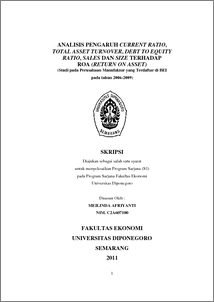# ANALISIS PENGARUH CURRENT RATIO, TOTAL ASSET TURNOVER, DEBT TO EQUITY RATIO, SALES DAN SIZE TERHADAP ROA (RETURN ON ASSET) (Studi pada Perusahaan Manufaktur yang Terdaftar di BEI pada tahun 2006-2009)

AFRIYANTI, Meilinda and CHABACHIB, Moch. (2011) ANALISIS PENGARUH CURRENT RATIO, TOTAL ASSET TURNOVER, DEBT TO EQUITY RATIO, SALES DAN SIZE TERHADAP ROA (RETURN ON ASSET) (Studi pada Perusahaan Manufaktur yang Terdaftar di BEI pada tahun 2006-2009). Undergraduate thesis, Universitas Diponegoro.Preview
PDF - Published Version
320Kb

## Abstract

Capital market is an indicator of a country's economic progress and to support economic development the country concerned.Various roles of capital markets in a country that is as a facility to do the interaction between buyers and sellers to measure the efficiency of company operations. One analytical tool in measuring the effectiveness of the company to generate profits is the Return on Assets (ROA). This study aims to examine the factors Current Ratio (CR), Total Asset Turnover (TATO), Debt to Equity Ratio (DER), Sales and Size of Return on Assets (ROA) at the manufacturing companies listed in Indonesia Stock Exchange period 2006 -2009. This study uses the entire population of manufacturing companies listed in Indonesia Stock Exchange (BEI) in 2006 to 2009, 151 companies. Sampling was done by purposive sampling technique.With the sampling method is derived samples used in this study as many as 17 companies. This study uses secondary data obtained from the publication of Indonesian Capital Market Directory (ICMD).Analytical techniques used in this study is the technique of multiple regression analysis with least squares equation and test the hypothesis F-statistic to test the effect together with a confidence level of 5% and using the t-statistic for testing the partial regression coefficients. In the classical assumption test results showed that there were no irregularities classical assumptions, this suggests that the available data has been qualified to use multiple linear regression model. Conclusions of this study were obtained as follows: Current Ratio variable is negative and significant impact on ROA, Total Asset Turnover variable has positive and significant impact on ROA, the variable Debt to Equity Ratio is negative and significant impact on ROA, Sales variable and not significant positive effect on ROA , and the Size variable is not significant positive effect on ROA. Regression equation obtained is ROA = 1.660 to 5.142 + 7.557 TATO - DER 5.408 + 0.274 + 0.616 SIZE SALES. Magnitude of the coefficient of determination (adjusted R²) is 32.5% which means 32.5% ROA is affected by independent variables, while the remaining balance of 67.5% is explained by other variables not presented in the study.

Item Type: Thesis (Undergraduate) Current Ratio (CR), Total Asset Turnover (TATO), Debt to Equity Ratio (DER), Sales, Size and Return on Assets (ROA). Current Ratio (CR), Total Asset Turnover (TATO), Debt to Equity Ratio (DER), Sales, Size and Return on Assets (ROA). H Social Sciences > H Social Sciences (General) Faculty of Economics and Business > Department of Management 29473 Mr. Perpustakaan Fakultas Ekonomi 26 Sep 2011 08:59 26 Sep 2011 08:59

Repository Staff Only: item control page